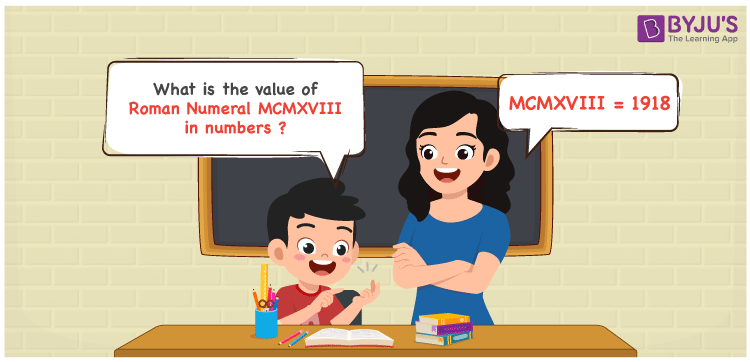Checkout JEE MAINS 2022 Question Paper Analysis : Checkout JEE MAINS 2022 Question Paper Analysis :

# MCMXVIII Roman Numerals

MCMXVIII Roman Numerals is 1918. The system of expressing numbers using the Roman alphabet is known as Roman Numerals. Students are advised to download the Roman Numerals in PDF format anytime to grasp the basic rules of expressing Roman letters accurately. In this article, we will discuss how to represent the number form of the Roman Numeral MCMXVIII.

 Number Roman Numeral 1918 MCMXVIII

## How to Write MCMXVIII Roman Numerals?We use the following methods to represent the numerical value of MCMXVIII Roman Numerals

First Method:

• Break the Roman Numerals into single letters
• MCMXVIII = M + (M – C) + X + V + I + I + I
• Write the numerical value of each letter and add/subtract them
• MCMXVIII = 1000 + (1000 – 100) + 10 + 5 + 1 + 1 + 1 = 1918

Second Method:

In this method, we consider the groups of Roman Numerals for addition or subtraction

• MCMXVIII = M + CM + X + VIII = 1000 + 900 + 10 + 8 = 1918
• Therefore the numerical value of MCMXVIII Roman Numerals is 1918

## Video Lesson on Roman Numerals## Related Articles

Roman Numerals 1 to 50

Roman Numerals 1 to 100

Roman Numerals 1 to 1000

Roman Numerals Conversion

L Roman Numerals

Roman Numeral XXXIX

## Frequently Asked Questions on MCMXVIII Roman Numerals

### What is the numerical value of MCMXVIII Roman Numerals?

The numerical value of MCMXVIII Roman Numerals 1918.

### What is the value of (99 – 88) + 1918 in Roman Numerals?

(99 – 88) + 1918 = 1929. The number 1929 in Roman Numerals is MCMXXIX. Thus the value of (99 – 88) + 1918 in Roman Numerals is MCMXXIX.

### How do you read the Roman Numeral MCMXVIII in English?

MCMXVIII Roman Numeral is equal to 1918 and it is read as One Thousand Nine Hundred and Eighteen in English.